4.9.3.2 Starting at the First Node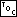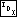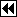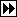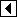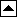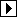To initialize a traverser to start at the least valued node, we simply descend from the root as far down and left as possible, recording the parent pointers on the stack as we go. If the stack overflows, then we balance the tree and start over.

```65. <BST traverser least-item initializer 65> =
void *bst_t_first (struct bst_traverser *trav, struct bst_table *tree) {
struct bst_node *x;

assert (tree != NULL && trav != NULL);

trav->bst_table = tree;
trav->bst_height = 0;
trav->bst_generation = tree->bst_generation;

x = tree->bst_root;
if (x != NULL)
while (x->bst_link != NULL)       {
if (trav->bst_height >= BST_MAX_HEIGHT)           {
bst_balance (tree);
return bst_t_first (trav, tree);
}

trav->bst_stack[trav->bst_height++] = x;